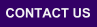Mplus
Tuesday
April 20, 2021Mplus Mplus at a GlanceGeneral DescriptionMplus Programs Pricing Version History System Requirements Platforms FAQ
 Analyses/ResearchMplus Examples Papers References
 Search

MODELING WITH CONTINUOUS LATENT VARIABLES

Ellipse A describes models with only continuous latent variables. Following are models in Ellipse A that can be estimated using Mplus:

• Regression analysis
• Path analysis
• Exploratory factor analysis
• Confirmatory factor analysis
• Structural equation modeling
• Growth modeling
• Discrete-time survival analysis
• Continuous-time survival analysis

Observed outcome variables can be continuous, censored, binary, ordered categorical (ordinal), unordered categorical (nominal), counts, or combinations of these variable types.

Special features available with the above models for all observed outcome variables types are:

• Single or multiple group analysis
• Missing data under MCAR, MAR, and NMAR and with multiple imputation
• Complex survey data features including stratification, clustering, unequal probabilities of selection (sampling weights), subpopulation analysis, replicate weights, and finite population correction
• Latent variable interactions and non-linear factor analysis using maximum likelihood
• Random slopes
• Individually-varying times of observations
• Linear and non-linear parameter constraints
• Indirect effects including specific paths
• Maximum likelihood estimation for all outcomes types
• Bootstrap standard errors and confidence intervals
• Wald chi-square test of parameter equalities
• Plausible values for latent variables